# Math Operations

Math operations are performed by several nodes. While some nodes are specialized (goniometric function, LowHigh filter), some functionalities overlap. For example addition is performed by Join as well as by Matrix nodes. We now describe major functionalities of these nodes and their usage.

## Matrix node

Purpose of this node is to simplify vanilla matrix operations such as addition, or multiplication.

### List of features

• The node allows a number of operations (like multiplication or addition) with a variety of inputs. For example matrix multiplication ($\mathbf{A} \cdot \mathbf{B}$, where $\mathbf{A}$,$\mathbf{B}$ are matrices), vector times matrix ($\mathbf{v}^{\mathsf{T}} \cdot \mathbf{A}$, where $\mathbf{v}$ is a vector), multiplication where inputs are vectors, ($\mathbf{w}^{\mathsf{T}} \cdot \mathbf{v}$, $\mathbf{v} \cdot \mathbf{w}^{\mathsf{T}}$), or operations with numbers like $c \cdot \mathbf{A}$ and much more.
• Two input types are supported for several operations: 1) a memory block from another node; 2) a number in the task property Execute/Params/DataInput.
• The Matrix Node is a layer above the MatrixAutoOps class, so you can use it in your code as well.

### Operations

The node always expects one input as a Memory Block (MB), A, and if second input is required, the second one goes to B (or it is optionally a number).

Operation Input Comment
Multiplication
$\mathbf A \cdot \mathbf B$
Two MB Each input can be a matrix, vector, or number.
Multiplication
$\mathbf A \cdot$DataInput0
One MB + DataInput0 MB input that goes into $\mathbf A$ can be matrix, vector, or number.
$\mathbf A + \mathbf B$
Two MB Each input can be matrix, vector, or number. If matrix/vector is used as input, the node performs row/column-wise addition.
$\mathbf A +$ DataInput0
One MB + DataInput0 MB input that goes into $\mathbf A$ can be matrix, vector, or number.
Substraction
$\mathbf A - \mathbf B$
Two MB Each input can be matrix, vector, or number. If matrix/vector is used as input, the node performs row/column-wise addition.
Substraction
$\mathbf A -$ DataInput0
One MB + DataInput0 MB input that goes into $\mathbf A$ can be matrix, vector, or number.
MultiplElemntWise
$\mathbf A \circ \mathbf B$
Two MB Element-wise product, each input can matrix, vector, or number.
DotProd
$\mathbf A^{\mathsf T} \cdot \mathbf B$
Two MB Each input is a vector. This operation can be additionally performed by the Multiplication operation.
MinIndex
$\underset{i}{\textrm{arg min}} ~\mathbf A_i$
One MB Returns the index of the min value in the abs of vector.
MaxIndex
$\underset{i}{\textrm{arg max}} ~\mathbf A_i$
One MB Returns the index of the max value in the abs of vector.
GetCol
$\mathbf A_{i,:}$
Two MB First MB input is matrix, second input is number that defines the column to get (first column has index 0).
GetCol
$\mathbf A_{i,:}$
One MB + DataInput0 MB input is matrix, DataInput0 defines the column to get (first one has index 0).
GetRow
$\mathbf A_{:,i}$
Two MB First MB input is matrix, second input is number that defines the row to get (first row has index 0).
GetRow
$\mathbf A_{:,i}$
One MB + DataInput0 MB input is matrix, DataInput0 defines the row to get (first one has index 0).
Minus
$-\mathbf A$
One MB MB input is matrix.
Norm2
$\Vert \mathbf A \Vert_2$
One MB Returns Norm2 of the input MB.
Normalize
$\frac{1}{\Vert \mathbf A \Vert_2} \mathbf A$
One MB Normalizes the input MB.
Exp, Log, Abs, Round, Floor, Ceil One MB Performs the desired operation on each element in the input MB.
Pow
Two MB Each input can be matrix, vector, or number. If matrix/vector is used as input, the node performs row/column/element-wise power operation.
Pow
One MB + DataInput0 MB input that goes into first input can be matrix, vector, or number.

### Using as a node in Brain Simulator

Here are few representative examples how to use the MatrixNode, it gives basic idea how to use the node and what it should do. More examples can be found in the Sample Projects, where you can play with parameters and copy-paste nodes directly into your projects.

Multiplication of two matrices

Two memory blocks that represent matrices are multiplied, the observers below show what is inside of the memory blocks.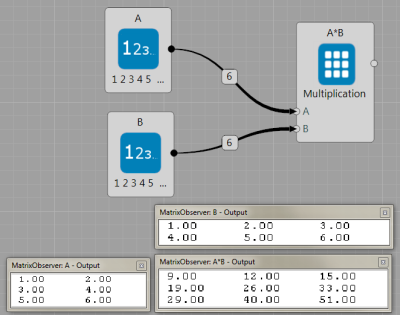Multiplication of vector with a matrix

Two memory blocks that represent a matrix and a vector are multiplied, the observers below again show what is inside of the memory blocks.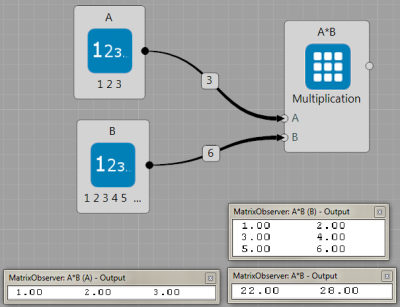Row-wise addition of matrix with a vector

Two memory blocks that represent a matrix and a vector are summed. The algorithm cannot perform element-wise addition because of the memory block sizes, but the number of columns of the matrix and the vector correspond. Thus the algorithm performs element-wise addition for each row.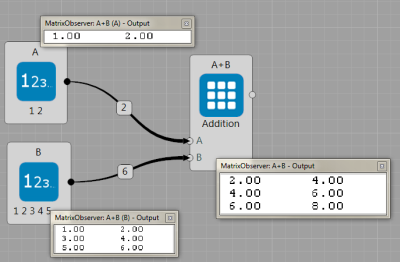Addition of a matrix with a constant number

The matrix node allows user to insert the constant as DataInput0 (see orange circle) and the values in the memory block A will be increased by it, as shown in the figure below. So the input vector $[1,2]$ becomes: $[1,2]+5=[6,7]$.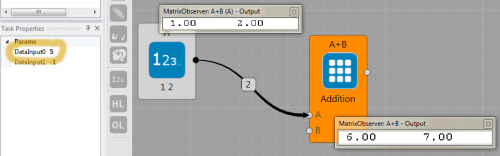### Using Matrix operations in C# code

You need to create the Matrix object

MyMatrixAutoOps mat_operation;


In the Init part, it is necessary to create an instance of the object and set-up the desired operations:

mat_operation = new MyMatrixAutoOps(Owner, Matrix.MatOperation.Multiplication | Matrix.MatOperation.Addition, A);


Inside, the details of the architecture such as the cublas library, CPU implementations, or kernel calls are hidden. So, you can directly multiply memory blocks A and B and save the result into memory the block C,

mat_operation.Run(Matrix.MatOperation.Multiplication, A, B, C);


or multiply A with number 10.4,

mat_operation.Run(Matrix.MatOperation.Multiplication, A, 10.4f, C);


Because the Addition operation was initialized too, the user can proceed,

mat_operation.Run(Matrix.MatOperation.Addition, A, B, C);


Complete code in the Brain Simulator node will look like:

public class MyExecuteTask : MyTask<MyNode>
{
private MyMatrixAutoOps mat_operation;

public override void Init(int nGPU)
{
mat_operation = new MyMatrixAutoOps(Owner, Matrix.MatOperation.Multiplication | Matrix.MatOperation.Addition, Owner.A);
}
public override void Execute()
{
mat_operation.Run(Matrix.MatOperation.Multiplication, Owner.A, Owner.B, Owner.C); // C = A*B
mat_operation.Run(Matrix.MatOperation.Multiplication, Owner.C, 10.3f, Owner.B); // B = C*10.3 = A*B*10.3
mat_operation.Run(Matrix.MatOperation.Addition, Owner.A, Owner.B, Owner.C);  // C = A+A*B*10.3
}
}


## LowHigh Filter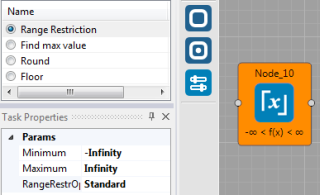Node to restrict the range of each element of the input memory block. There are two methods to apply:

• Standard: simply cuts all values higher or lower.

• Modulo: applies the modulus operator that computes the remainder from the integer division. So the result is: $\text{value} ~\%~ \text{Maximum} + \text{Minimum}$

## Reduction

The purpose of the Reduction node is to scale down the input memory block. As it efficiently operates on the cuda using the binary operations, the input is often re-casted, so even if integer is expected, user can provide float too.

### Operations

The node always expects one input as a Memory Block (MB) A, and if second input is required, the second one goes to B (or it is optionally a number).

Operation Input Output Comment
i_Sum_i integer MB integer sum the input
i_MinIdx_2i integer MB two integers min value and its index
i_MaxIdx_2i integer MB two integers max value and its index
i_MinIdxMaxIdx_4i integer MB four integers min value, its index, max value and its index
f_Sum_f float MB float sum of input
f_MinMax_2f float MB two floats min value and max value of the input
f_DotProduct_f float MB (vector) float dot product